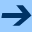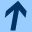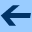Higher-order methods of collections

# Higher-order methods of collections

When working with collections, such as arrays or lists, we often use for-loops to perform some operation on all the elements of the collection:

>>> val C = listOf("Introduction to Programming",
...                "Programming Practice",
...                "Data Structures",
...                "Programming Principles",
...                "Algorithms",
...                "Programming Languages")
>>> C
[Introduction to Programming, Programming Practice, Data Structures, Programming Principles, Algorithms, Programming Languages]
>>> for (e in C)
...   println(e)
Introduction to Programming
Programming Practice
Data Structures
Programming Principles
Algorithms
Programming Languages


The interesting part of this loop is the print statement. Modern programming languages make it possible to write code that concentrates on this part, not on the loop.

Instead of writing a for-loop, we can use the forEach method of the collection. This is a higher-order method, that is, it takes a function object as its argument.

The loop above is changed to this:

>>> C.forEach { s: String -> println(s) }
Introduction to Programming
Programming Practice
Data Structures
Programming Principles
Algorithms
Programming Languages


As we learnt in the previous section, this can be simplified a bit, because the Scala compiler knows that the argument of forEach has to be a function object of type (String) -> Unit. Therefore we are allowed to omit the type of the argument.

>>> C.forEach { s -> println(s) }
Introduction to Programming
Programming Practice
Data Structures
Programming Principles
Algorithms
Programming Languages


Furthermore, there is only a single parameter, so we can replace it by the magic parameter name it.


>>> C.forEach { println(it) }
Introduction to Programming
Programming Practice
Data Structures
Programming Principles
Algorithms
Programming Languages


Here is another example where we use forEach to compute the sum of the elements of a collection:

>>> val l = listOf(15, 39, 22, 98, 37, 19, 5)
>>> l
[15, 39, 22, 98, 37, 19, 5]
>>> var sum = 0
>>> l.forEach { sum += it }
>>> sum
235


Again we used the shortcut syntax we used for the function literal x: Int -> sum += x.

#### Any, all, count, and find

Often one goes through the elements of a collection to determine one of the following properties:

• Does the collection contain an element with a certain property?
• Do all the elements have the property?
• How many of the elements have the property?
• Find an element with the property.

This can be done nicely using the higher-order methods any, all, count, and find. They all require a function object that maps the elements to Boolean.

Here are some examples:

>>> val C = listOf("Introduction to Programming",
...                "Programming Practice",
...                "Data Structures",
...                "Programming Principles",
...                "Algorithms",
...                "Programming Languages")
>>> C.count { "Programming" in it }
4
>>> C.count { "Programming" !in it }
2
>>> C.all { " " in it }
false
>>> C.any { " " !in it }
true
>>> C.any { "Algo" in it }
true
>>> C.all { "A" in it.toUpperCase() }
true
>>> C.find { " " !in it }
Algorithms


#### Filtering a collection

Often we want to pick a subset of a collection consisting of all the elements that satisfy a certain condition. The higher-order methods filter and filterNot can do this. Again, they take as argument a function object that maps an element to a Boolean.

Here are some basic examples, using the list from above:

>>> C.filter { " " in it }
[Introduction to Programming, Programming Practice, Data Structures, Programming Principles, Programming Languages]
>>> C.filterNot { " " in it }
[Algorithms]


Note that filterNot does the same as filter, but reverses the sense of the condition.

Here are some interesting filters using our file of 113809 English words:

>>> val words = java.io.File("words.txt").useLines { it.toSet() }
>>> words.filter { "issis" in it }
[missis, missises, narcissism, narcissisms, narcissist, narcissists]
>>> words.filter { it.length > 20 }
[counterdemonstrations, hyperaggressivenesses, microminiaturizations]
>>> words.filterNot { "a" in it || "e" in it || "o" in it || "u" in it || "i" in it }
[by, byrl, byrls, bys, crwth, crwths, cry, crypt, crypts, cwm, cwms,
cyst, cysts, dry, dryly, drys, fly, flyby, flybys, flysch, fry,
ghyll, ghylls, glycyl, glycyls, glyph, glyphs, gym, gyms, gyp, gyps,
gypsy, hymn, hymns, hyp, hyps, lymph, lymphs, lynch, lynx, my,
myrrh, myrrhs, myth, myths, nth, nymph, nymphs, phpht, pht, ply,
pry, psst, psych, psychs, pygmy, pyx, rhythm, rhythms, rynd, rynds,
sh, shh, shy, shyly, sky, sly, slyly, spry, spryly, spy, sty, stymy,
sylph, sylphs, sylphy, syn, sync, synch, synchs, syncs, syzygy, thy,
thymy, try, tryst, trysts, tsk, tsks, tsktsk, tsktsks, typp, typps,
typy, why, whys, wry, wryly, wych, wynd, wynds, wynn, wynns, xylyl,
xylyls, xyst, xysts]


Filtering is the essence of the Sieve of Erathosthenes, so it is natural that we can write it concisely using filter (primes.kts):

val n = args.toInt()
val sqrtn = Math.sqrt(n.toDouble()).toInt()

var s = (2 .. n).toList()

while (true) {
val k = s.first()
if (k > sqrtn)
break
print("\$k ")
s = s.filter { it % k != 0 }
}

println(s.joinToString(separator=" "))


#### Transforming a collection

Another common operation is to create a new collection by somehow transforming every element of the existing collection.

This can be done using the higher-order function map. Its argument is a function object that maps the elements of the collection to its transformed value. The new collection can have the same or a different type:

>>> C
[Introduction to Programming, Programming Practice, Data Structures, Programming Principles, Algorithms, Programming Languages]
>>> C.map { it.length }
[27, 20, 15, 22, 10, 21]
>>> C.map { it.toUpperCase() }
[INTRODUCTION TO PROGRAMMING, PROGRAMMING PRACTICE, DATA STRUCTURES,
PROGRAMMING PRINCIPLES, ALGORITHMS, PROGRAMMING LANGUAGES]
>>> C.map { it + " " + it }
[Introduction to Programming Introduction to Programming, Programming
Practice Programming Practice, Data Structures Data Structures,
Programming Principles Programming Principles, Algorithms
Algorithms, Programming Languages Programming Languages]


#### Sorting a collection

We have already seen that we can sort a list by using its sorted method. This always sorts the elements using the natural ordering of the element type.

If we want to sort with a different ordering, we can use the higher-order methods sortedWith (which returns a new list) or sortWith (which sorts the given mutable list). They take as an argument a java.util.Comparator object to handle the comparison between elements of the list.

We can create a Comparator object from a function object that maps two elements $$a$$ and $$b$$ to an Int. The function should return a negative integer if element $$a$$ should appear before element $$b$$ in the desired sorted order, a positive integer if $$a$$ should appear after $$b$$, and zero if $$a$$ and $$b$$ are considered equal in the sorted order.

Here is an example:

>>> val words = java.io.File("words.txt").useLines { it.toSet() }
>>> words.sortedWith(java.util.Comparator { a: String, b: String -> b.length - a.length }).take(10)
[counterdemonstrations, hyperaggressivenesses, microminiaturizations,
counterdemonstration, counterdemonstrators, hypersensitivenesses,
microminiaturization, representativenesses, anticonservationist,
comprehensivenesses]


It sorts the elements of the words list in order of decreasing length (and then we display only the first 10 elements).

Note that every comparable type already has a method compareTo(a, b), which returns a positive integer if $$a > b$$, a negative integer if $$a < b$$, and zero if $$a = b$$:

>>> 17.compareTo(19)
-1
>>> 17.compareTo(13)
1
>>> 17.compareTo(17)
0
>>> "CS109".compareTo("Otfried")
-12


We can make use of this when building our own Comparator objects, for instance like this:

>>> words.sortedWith(java.util.Comparator<String> { a, b -> -a.length.compareTo(b.length) }).take(10)
[counterdemonstrations, hyperaggressivenesses, microminiaturizations,
counterdemonstration, counterdemonstrators, hypersensitivenesses,
microminiaturization, representativenesses, anticonservationist,
comprehensivenesses]


Actually, we can do this even easier: There are methods sortedBy and sortBy that take a function object that maps the elements of the list to some other, comparable type R. The list is then sorted by the order on R:

>>> words.sortedBy { -it.length }.take(10)
[counterdemonstrations, hyperaggressivenesses, microminiaturizations,
counterdemonstration, counterdemonstrators, hypersensitivenesses,
microminiaturization, representativenesses, anticonservationist,
comprehensivenesses]


We have sorted the words by their negative length, so the longest word comes first. If you find the negation a bit unlogical, you can instead write it like this:

>>> words.sortedByDescending { it.length }.take(10)
[counterdemonstrations, hyperaggressivenesses, microminiaturizations,
counterdemonstration, counterdemonstrators, hypersensitivenesses,
microminiaturization, representativenesses, anticonservationist,
comprehensivenesses]

This is actually longer, but perhaps easier to understand.

#### More higher-order methods

Kotlin collections have many more higher-order methods. Here are few examples:

findLast finds the last element in a list satisfying the given property (remember that find would find the first element):

>>> val l = listOf(13, 7, 2, 19, 20, 1, 17, 35, 9)
>>> l.find { it > 15 }
19
>>> l.findLast { it > 15 }
35


retainAll is like filter, but it modifies the MutableList itself, throwing away all elements that do not satisfy the property (while filter always returns a new list):

>>> val l = mutableListOf(13, 7, 2, 19, 20, 1, 17, 35, 9)
>>> l.filter { it > 15 }
[19, 20, 17, 35]
>>> l
[13, 7, 2, 19, 20, 1, 17, 35, 9]
>>> l.retainAll { it > 15 }
true
>>> l
[19, 20, 17, 35]


takeWhile and takeLastWhile return a new list with the elements from the beginning (or the end) of the given list that satisfy a property. Similary, dropWhile and dropLastWhile return the remaining elements:

>>> val l = listOf(13, 7, 2, 19, 20, 1, 17, 35, 9)
>>> l.takeWhile { it > 5 }
[13, 7]
>>> l.dropWhile { it > 5 }
[2, 19, 20, 1, 17, 35, 9]
>>> l.takeLastWhile { it > 5 }
[17, 35, 9]
>>> l.dropLastWhile { it > 5 }
[13, 7, 2, 19, 20, 1]


groupBy is a useful operation: It applies a function object to every element of the collection to obtain a "key" for that element. It then returns a map that maps these keys to lists of the original elements:

>>> val l = listOf(13, 7, 2, 19, 20, 1, 17, 35, 9)
>>> l.groupBy { it % 3 }
{1=[13, 7, 19, 1], 2=[2, 20, 17, 35], 0=}
>>> val m = words.groupBy { it.length }
>>> m
[counterdemonstration, counterdemonstrators, hypersensitivenesses, microminiaturization, representativenesses]
>>> m
[counterdemonstrations, hyperaggressivenesses, microminiaturizations]
>>> m
null


flatMap is a very powerful operation: It applies a given function object to every element of the collection. The result of the function object should be a list (or set, or array). The result of flatMap is a list combining all these lists in the order of the original list.

fold and reduce or other powerful operations that go a bit beyond the scope of this section. Learn about them later!Higher-order methods of collections